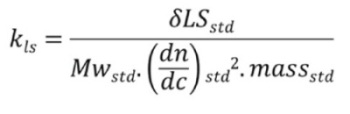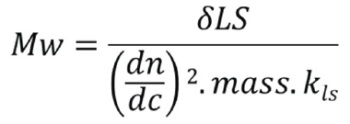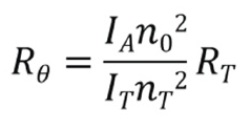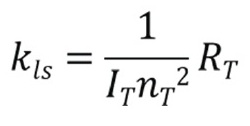# Calibration of Static Light Scattering Measurements

Light scattering methods are often called ‘'absolute’ because the addition of a light scattering detector to an SEC system enables direct molecular weight measurement without creating a calibration curve.

This theory is derived from first principles without any assumptions, but nevertheless, at some point in the process, it becomes necessary to relate the scattering light intensity to the molecular weight of the molecule scattering the light. This necessitates some sort of instrument calibration, or the creation of response factors. In this respect, the method cannot really be called truly 'absolute'.

## Principle of Instrument Calibration

Although calibration is not required for the column, all light scattering instruments need to be calibrated in some form, and can be calibrated via many different methods. The principle of the light scattering calibration is to relate the size of the response signal to the parameter under measurement.

In static light scattering, the magnitude of the signal obtained from the photo detector is related to the scattering light intensity and to the sample’s molecular weight.

Besides all sample dependent factors expressed in the Rayleigh equation, the measured scattering light intensity will rely on the following instrument dependent variables:

• Detector sensitivity
• Incident laser power
• Scattering volume, which is the intersection volume of the laser beam and the detection optics
• The distance from the scattering volume to the detector
• Flare and refraction effects at the interface between the cell and the windows.

Since all these components cannot be characterized in a system to achieve the required measurement accuracy, they are all represented by a calibration step.

## Calibration Techniques

There are two different yet functionally identical techniques available for the calibration of a system: Molecular weight standard-based calibration and Scattering standard-based calibration.

## Molecular Weight Standard-Based Calibration

A molecular weight standard-based calibration involves a test run of a sample of known molecular weight in a static light scattering (SLS) detector coupled to a GPC/SEC system. Using the known sample concentration, the magnitude of the response of the SLS detector molecular weight and dn/dc, and discounting values such as the laser wavelength and solvent refractive index, a calibration constant or instrument response factor can be calculated for the SLS detector as defined by the following equation:Where,

Kls is the light scattering calibration constant,

δLSstd is measured intensity of light scattered by the standard,

Mwstd is the molecular weight of the standard,

dn/dc is the standard refractive index increment, and massstd is the injected mass of the standard.

The determination of a calibration constant enables the molecular weight measurement for any sample as defined the following equation:Where,

Mw is the molecular weight of the sample,

δLS is the measured scattering light intensity of the sample,

dn/dc is the sample refractive index increment,

mass is the injected mass of the sample, and Kls = the light scattering calibration constant.

## Advantages of Molecular Weight Standard-Based Calibration

The following are some advantages of molecular weight standard-based calibration:

• A traceable and well-defined standard enables simultaneous calibration of all detectors in a GPC/SEC system.
• This calibration step can account for the inter-detector volumes between the flow cells of different detectors and the band broadening and tailing taking place when the sample travels between the detectors.
• Allows for checking of calibration or re-calibration at any time

## Scattering Standard-Based Calibration

A scattering standard-based calibration involves the filling of the flow cell of a light scattering devicewith a solvent of known scattering, such as toluene, which has a well-defined Rayleigh ratio. Hence, the scattering signal from a sample can be calculated through the measurement of the scattering signal from toluene. Rθ for the sample is measured as defined in the following equation:Where,

Rθ is the sample Rayleigh ratio,

IA is the scattered light intensity from sample,

n0 is the refractive index of the sample solvent,

IT is the intensity of light scattered by toluene,

nT is the refractive index of toluene, and RT is the Rayleigh ratio of toluene.

By inputting the calculated Rθ value into the Rayleigh equation, the molecular weight of the sample can be calculated. In this respect, the calibration constant is defined as follows:## Drawbacks of Scattering Standard-Based Calibration

The following are the drawbacks of the scattering standard-based calibration:

• The refractive index of the scattering standard only allows the 90° detector to be calibrated, necessitating the normalization of all of the other detector responses (in a system consisting of more than one angle) against the 90° detector. This, in turn, necessitates the acquisition of normalization factors for the non-90° detectors by running a standard through the system.
• Inter-detector volumes or peak broadening or tailing cannot be exacted and thus must be carried out separately.
• This is a laborious process as the light scattering detector needs to be taken completely off-line.

## Conclusion

A molecular weight standard-based calibration enables simultaneous calculation of all of the instrument response factors/calibration constants as well as the inter-detector volumes and peak broadening parameters.

It is a more efficient calibration technique as it ensures the calibration of a system through the comparison of the responses of the standard at the time of calibration. A scattering standard-based calibration is comparatively laborious and does not advance the calibration, but rather only serves to add more obstacles to obtaining an accurate calibration of a system.This information has been sourced, reviewed and adapted from materials provided by Malvern Panalytical.

## Citations

• APA

Malvern Panalytical. (2019, September 03). Calibration of Static Light Scattering Measurements. AZoM. Retrieved on October 21, 2020 from https://www.azom.com/article.aspx?ArticleID=9823.

• MLA

Malvern Panalytical. "Calibration of Static Light Scattering Measurements". AZoM. 21 October 2020. <https://www.azom.com/article.aspx?ArticleID=9823>.

• Chicago

Malvern Panalytical. "Calibration of Static Light Scattering Measurements". AZoM. https://www.azom.com/article.aspx?ArticleID=9823. (accessed October 21, 2020).

• Harvard

Malvern Panalytical. 2019. Calibration of Static Light Scattering Measurements. AZoM, viewed 21 October 2020, https://www.azom.com/article.aspx?ArticleID=9823.